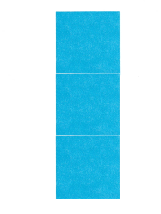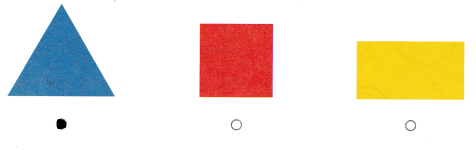# Texas Go Math Kindergarten Module 17 Assessment Answer Key

Refer to our Texas Go Math Kindergarten Answer Key Pdf to score good marks in the exams. Test yourself by practicing the problems from Texas Go Math Kindergarten Module 17 Assessment Answer Key.

## Texas Go Math Kindergarten Module 17 Assessment Answer Key

Concepts and Skills

Question 1.The name of the above shape is square with four sides and four vertices.

Question 2.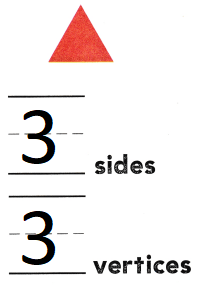The name of the shape is a triangle with three sides and three vertices.

Question 3.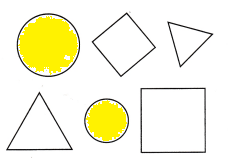There are 2 circles.

Question 4.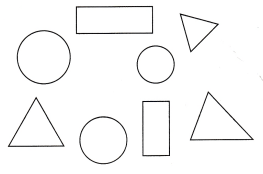There are 3 triangles in the above picture.

Directions
1-2 Trace around each shape, Write how many sides. Place a counter on each corner or vertex. Write how many vertices. TEKS K.6.D 3. Color all of the circles. TEKS K.6.A 4. Color all of the triangles. TEKS K.6.A

Question 5.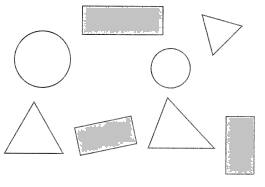There are 3 rectangles in the above picture.

Question 6.There are 2 squares in the above picture.

Question 7.